# Intersect

Intersect

Have you ever seen two roads crossing each other?

These roads would be intersecting each other.

The figure below shows intersecting roads.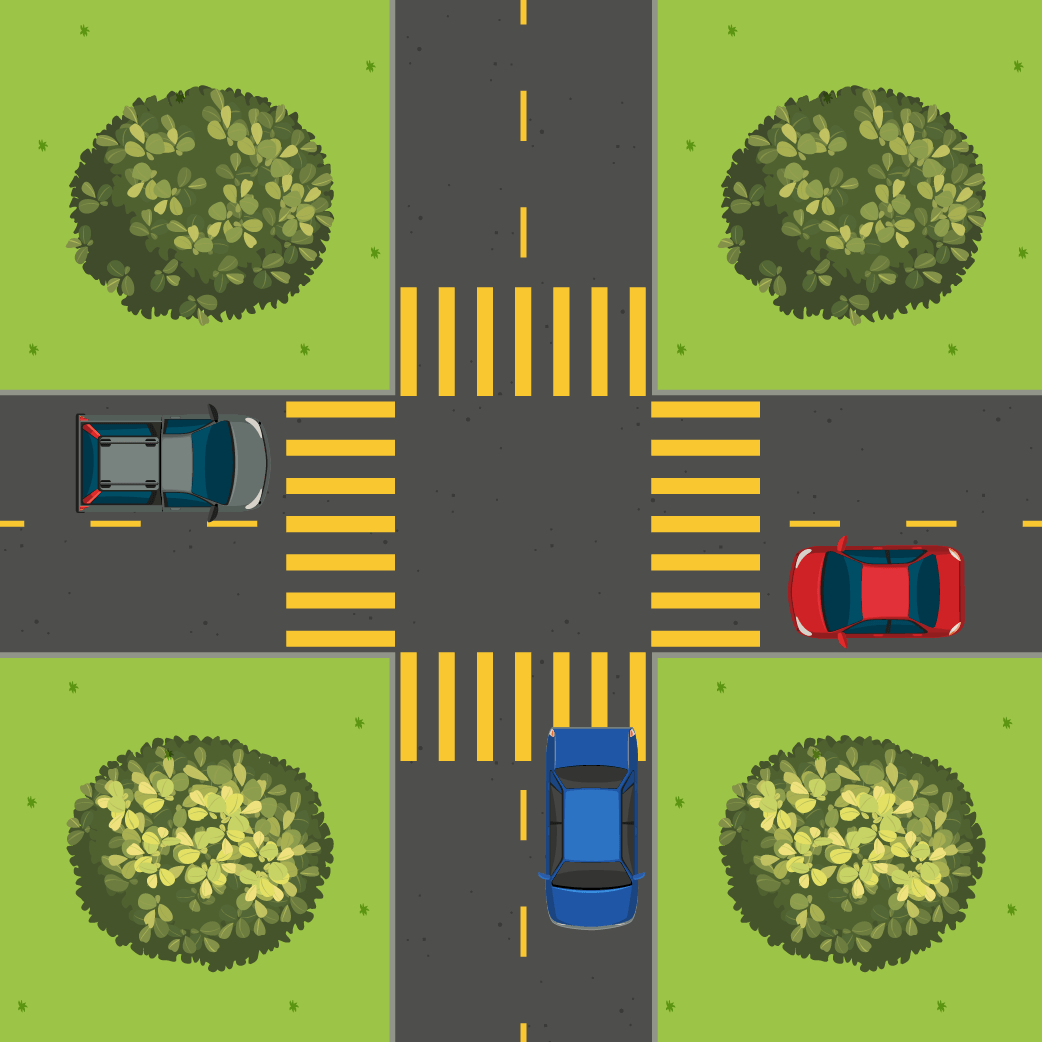The topic that we will be learning today in this mini-lesson deals with intersects.

## Lesson Plan

 1 What Is an Intersect? 2 Important Notes on Intersect 3 Solved Examples on Intersect 4 Challenging Questions on Intersect 5 Interactive Questions on Intersect

## What Is an Intersect?

Intersect definition: Intersects can be defined for lines and for sets.

When we talk about an intersect for a line, it would mean to divide by passing through or across something.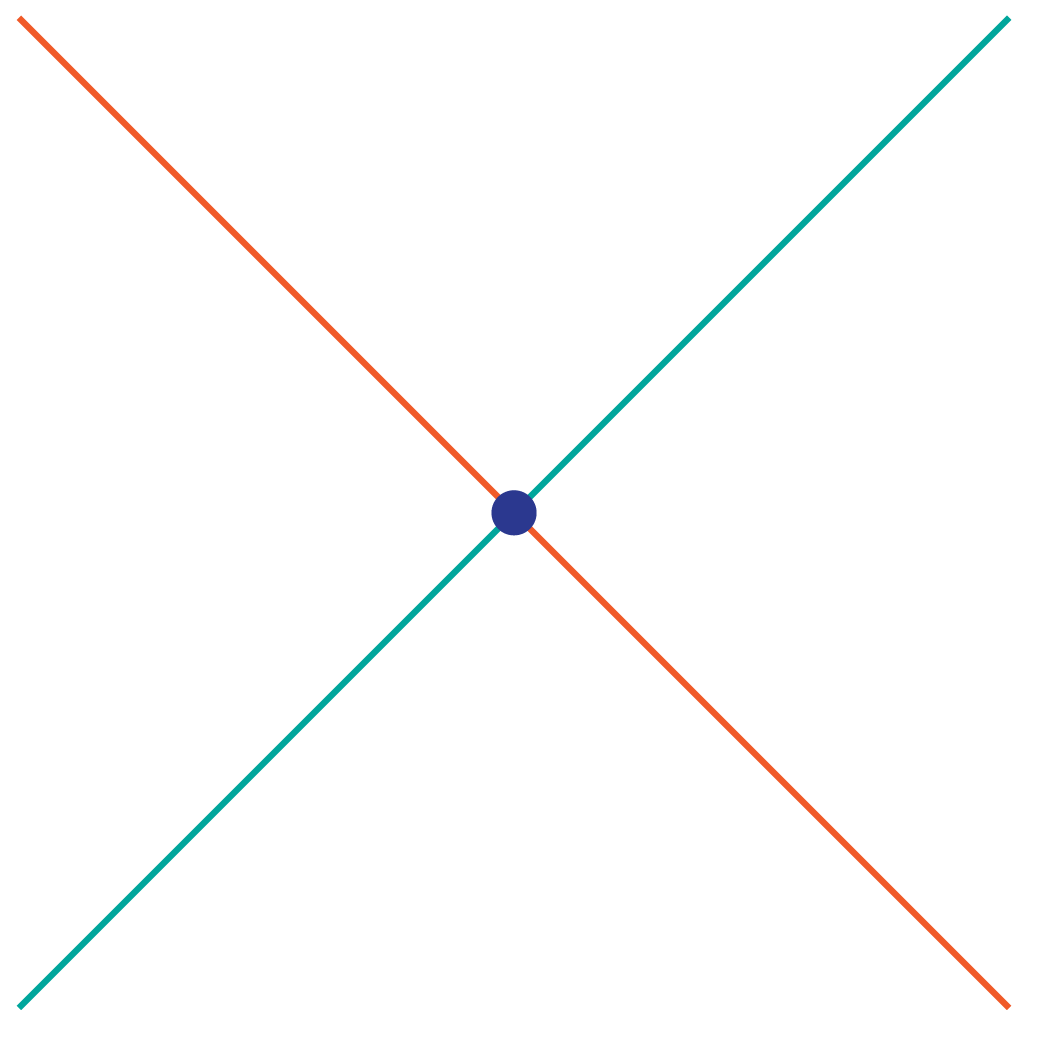However, when we talk about two sets, an intersect would mean if they have at least one element in common.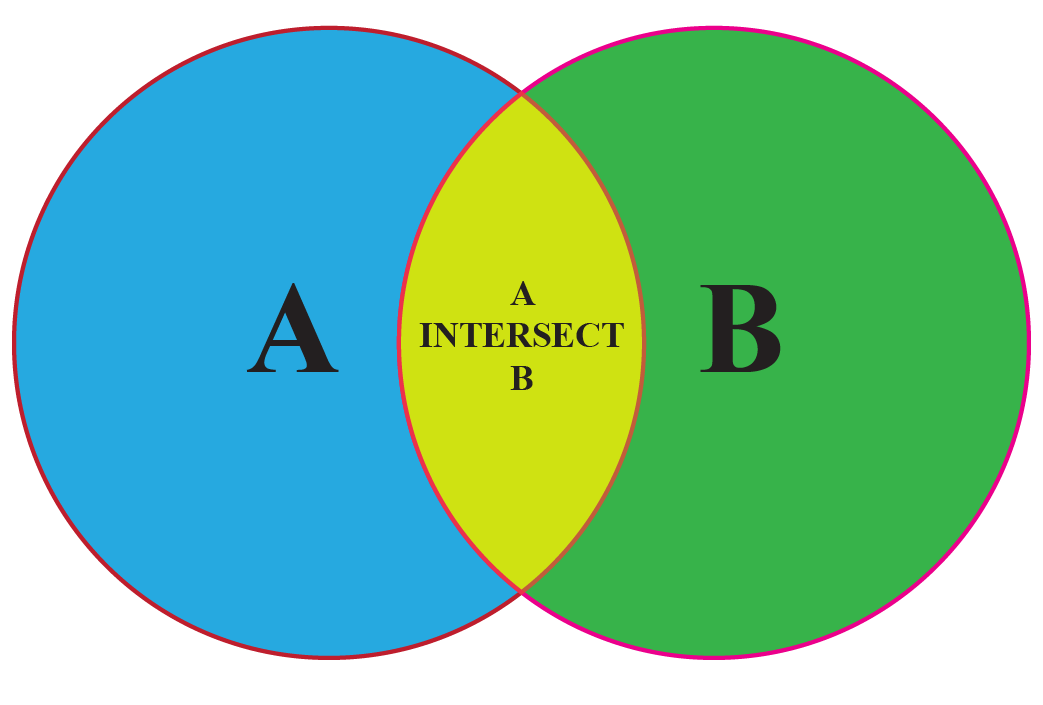Here is an interesting simulation to find the intersection of sets:

## What is Intersection of Lines?

When two lines or more than two lines cross each other in a plane, this is called the intersection of lines, and the lines are called intersecting lines.

They have a common point called the point of intersection.

In the figure shown below, we can see line P and line Q are intersecting at point O.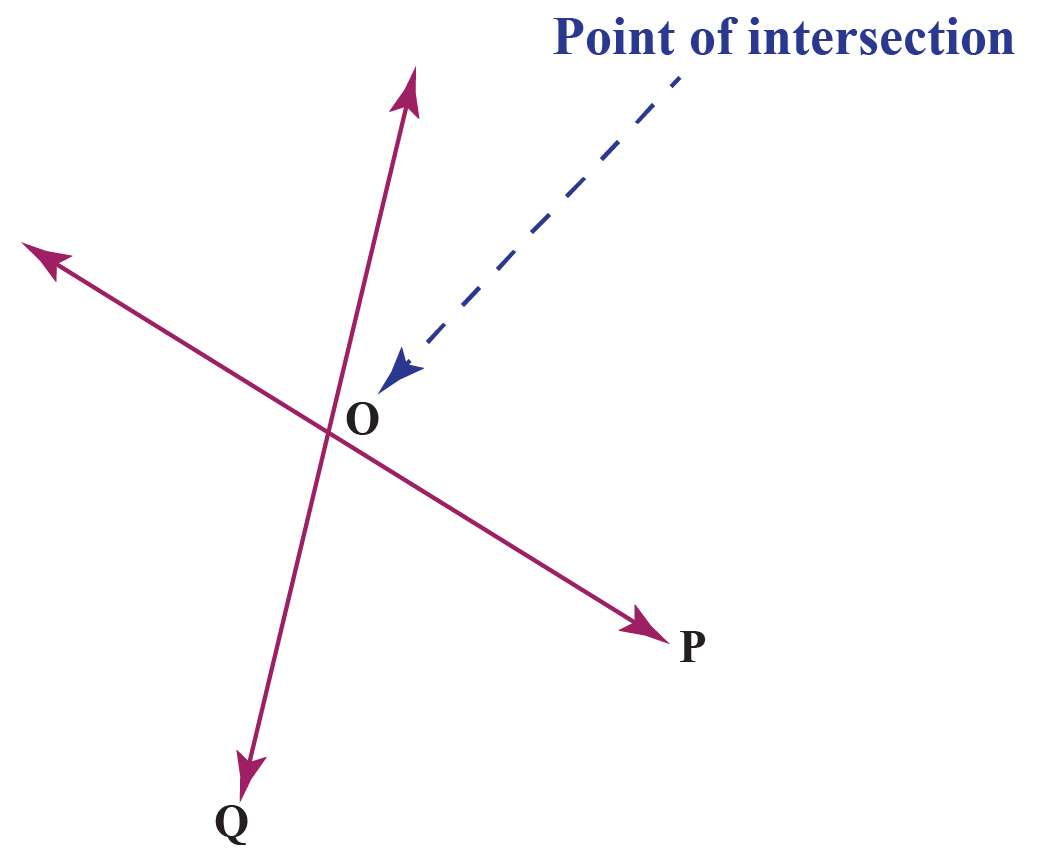### Cramer's Rule to Find the Point of Intersection

The Cramer's rule is used for solving linear equations.

We can use Cramer’s rule to find out the point of intersection:

\begin{align}\frac{x_{0}}{b_{1}c_{2}-b_{2}c_{1}}=\frac{-y_{0}}{a_{1}c_{2}-a_{2}c_{1}}=\frac{1}{a_{1}b_{2}-a_{2}b_{1}}\end{align}

## What Is Intersection of Sets?

The intersection of two sets says $$A$$ and $$B$$ is the set that contains all the elements of set $$A$$ that also belong to set $$B$$.

It is denoted as:

$$A$$ $$\cap$$ $$B$$

The below-given image shows two sets, $$A$$ and $$B$$, and their intersection.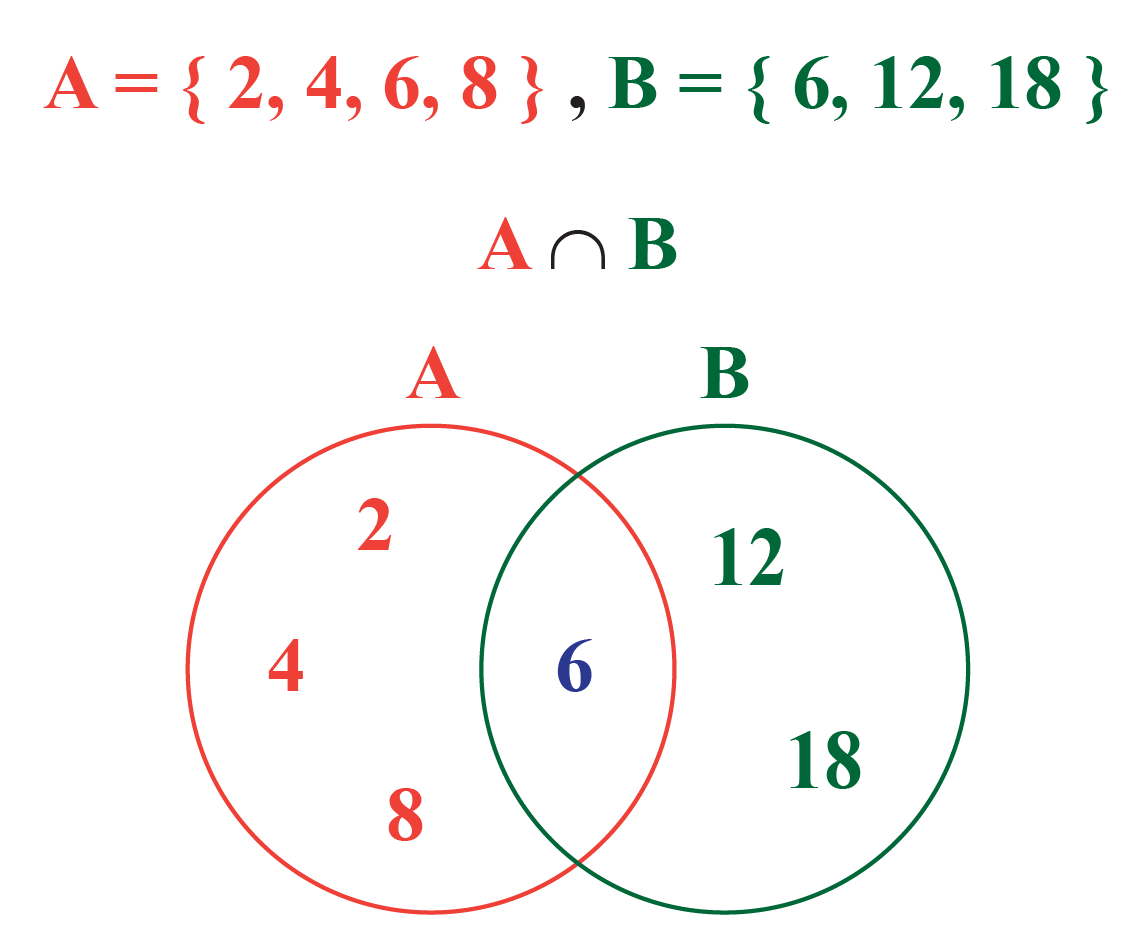## What Is the Intersection Symbol for Sets?

The intersection of sets is represented by the symbol $$\bigcap$$.

More Important Topics
More Important Topics